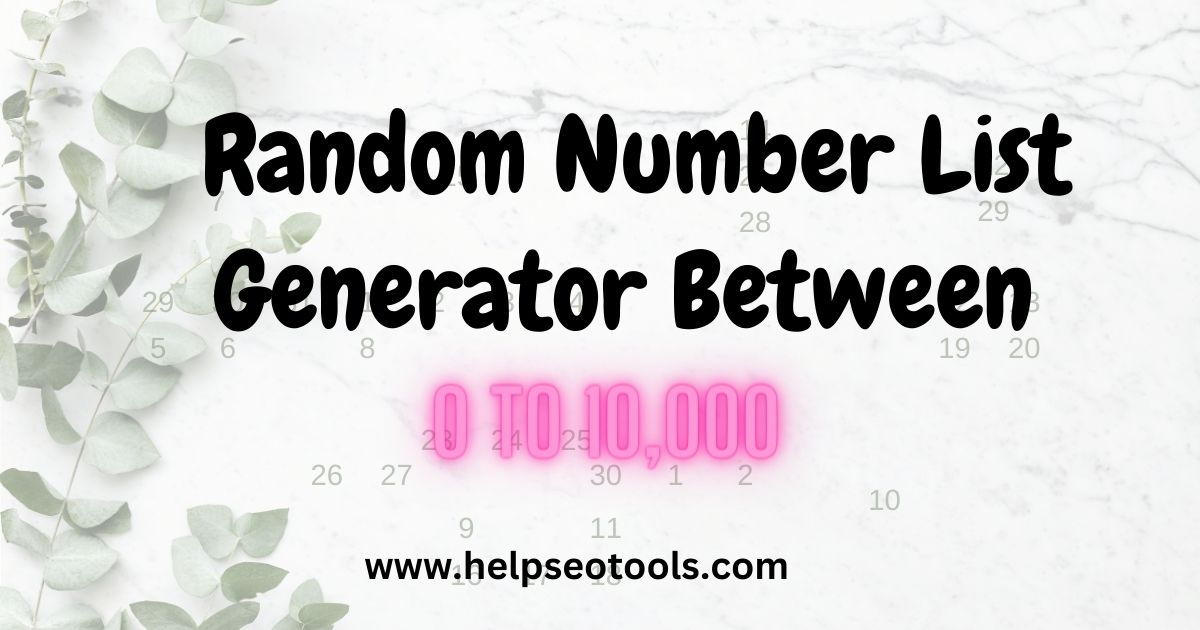# Random Number List Generator Between 1 and 10000 or more - No Repeats

Are you looking for a quick and easy way to generate unique random numbers list? then you are right place just a few clicks, you can generate random integers or decimal numbers within a specified range. To get started, simply enter the range of numbers you want to generate and click the "Generate" button. The tool will instantly produce a random number within that range. You can also choose to generate multiple numbers at once or copy and paste the results for later use.

Generate Random Number list Online Without Duplicates.

## About random number generator no-repeats tool?

First, let's start with the most basic type of random number generator, which is the one that generates a random number between two numbers range. This tool is useful when you need to generate a number within a specific range. For example, if you need to generate list of a random number between 1 and 100 or 1 and 10000, you can use this type of random number generator.

Another type of random number generator is the one that generates random numbers without repeats. This type of generator is useful when you need to randomly select items from a list without selecting the same item twice. For example, if you need to assign a unique number to each participant in a contest, you can use this type of random number generator to ensure that each number is unique.

#### Our Random Number Generator gives you several different options when you need to generate random numbers.

• How many
• Min and Max
• Sort
• Separator
• No Repeats

If you're looking for the best random number generator, there are many different options available on google. Some of the factors to consider when choosing a random number generator include the range of numbers available, the ability to generate random numbers without repeats, and the ability to customize the output.## How to us Random Number Generator 1-1000?

• Juat enter how many random numbers you want to generate. The minimum number can be 0 and the maximum is 10,000.
• then enter a minimum number and a maximum number. The randomly generated numbers will generate random number within this custom range. Make sure Min is lower than Max, or else you will encounter an error.
• Once you done click on generate button. You will get answer in few seconds. Now you can “Copy” button and “Ctrl+C” to copy the result to your clipboard.

Overall, the Random Number Generator on helpseotools is a convenient and reliable tool for anyone who needs to generate random numbers. Give it a try today and see how it can help you!

## Most Comman Random Number Generator from this tool is :

• Random Number Generator between 1 and 10
• Random Number Generator between 10 and 50
• Random Number Generator between 50 and 100
• Random Number Generator between 1 and 200
• Random Number Generator between 1 and 300
• Random Number Generator between 1 and 400
• Random Number Generator between 1 and 500
• Random Number Generator between 1 and 100
• Random Number Generator between 100 and 1000
• Random Number Generator between 1 and 1000
• Random Number Generator between 1 and 10000